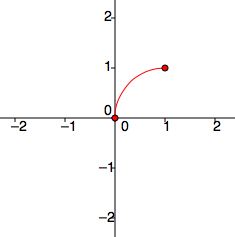### Home > PC > Chapter 11 > Lesson 11.1.1 > Problem11-10

11-10.

Make a table of at least eight values and then graph $r = 2 \cos θ$.

r

θ

$0$

$\frac { \pi } { 4 }$

$\sqrt 2$

$\frac { \pi } { 2 }$

$\frac {3 \pi } { 4 }$

r

θ

$\pi$

$\frac {5 \pi } { 4 }$

$0$

$\frac { 3\pi } { 2 }$

$\frac {7 \pi } { 4 }$

Add the rest of the points in your table to complete the graph.